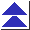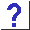# 14. Floating Point

14.1 When I set a float variable to, say, 3.1, why is printf printing it as 3.0999999?

14.2 I'm trying to take some square roots, and I've simplified the code down to

```	main()
{
printf("%f\n", sqrt(144.));
}
```
but I'm still getting crazy numbers.

14.3 I'm trying to do some simple trig, and I am #including <math.h>, but the linker keeps complaining that functions like sin and cos are undefined.

14.4a My floating-point calculations are acting strangely and giving me different answers on different machines.

14.4b I'm sure I've got the trig functions declared correctly, but they're still giving me wrong answers.

14.5 What's a good way to check for ``close enough'' floating-point equality?

14.6 How do I round numbers?

14.7 Why doesn't C have an exponentiation operator?

14.8 The predefined constant M_PI seems to be missing from my machine's copy of <math.h>.

14.9 How do I set variables to, or test for IEEE NaN (``Not a Number'') and other special values?

14.10 How can I handle floating-point exceptions gracefully?

14.11 What's a good way to implement complex numbers in C?

14.12 I'm looking for some code to do:

Fast Fourier Transforms (FFT's)
matrix arithmetic (multiplication, inversion, etc.)
complex arithmetic

14.13 I'm having trouble with a Turbo C program which crashes and says something like ``floating point formats not linked.''